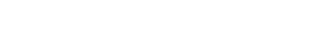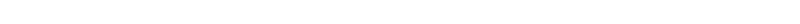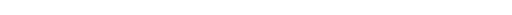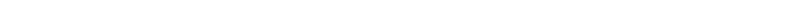# How to VLOOKUP from Another Sheet in Google Sheets?

When working with multiple datasets across worksheets in Google Sheets, it can get quite frustrating and time-consuming to look up data between different sheets.

Google sheets, however, provides a feature that saves you the trouble by looking up the data for you.

It looks up and retrieves matching data from another table, which could be on the same sheet or on a different sheet.

In this tutorial, I will show you how to use the VLOOKUP formula in Google Sheets and how to VLOOKUP from another sheet (from the same workbook or different workbook).

## What is the VLOOKUP Function In Google Sheets?

VLOOKUP stands for Vertical Lookup. It is a Google Sheets function that lets you perform a vertical lookup. In other words, it lets you search a particular lookup value down the first column of a range of cells.

Once it finds a matching value, it looks for a value in another specified column in the same row as the lookup value and retrieves it.

If this definition sounds confusing, hang in there. It will get clearer when we explain it with an example.

There is a notion among a lot of Google Sheets newbies that VLOOKUP is difficult to understand and apply.

However, this is not true.

In this tutorial, you’ll see how easy it is to apply. Once you get the hang of it you will also realize how powerful a tool it can be!

## When to Use VLOOKUP?

Let’s say you have two tables.

One that contains information about the employees and another that contains their total sales on a particular month.Both tables have a common column, the Employee ID. Moreover, the employee ID can be considered as a key-value or a unique identifier in both tables.

Say you need to know the Hourly Rate for each of the two employees featured in the Sales Table. These rates already exist in the Employee Table.

So, you need to pull the hourly rates corresponding to the two employee IDs from the Employee Table to the Sales Table.

Now you could just look for the two employee IDs in the Employee Table and copy their corresponding Hourly Rates into the Sales Table. But that would be really hard to do if you’re dealing with really large and complex datasets (which, in practice, is usually the case).

So, the best way to go around this is to use the VLOOKUP feature of Google Sheets.

In this tutorial, we will show you how to use VLOOKUP to solve the above issue, so that you can easily apply the function to your own data.

The syntax for VLOOKUP in Google sheets is as follows:

`VLOOKUP(search_key, range, index, [is_sorted])`

Here,

• search_key represents the unique identifier or key value that you want to look up. This can either be a value or a reference to a cell containing the value.
• range is the range of cells (in the source table) within which the VLOOKUP function should search. Always make sure that this range contains the column containing the search key as well as the column containing the corresponding value to be retrieved. VLOOKUP always searches the first column of range to find the search key.
• index is the column number within the range from which the corresponding value (the one in the same row as search_key) should be retrieved. So, the first column within the range has an index of 1, the second column has an index of 2, and so on.
• is_sorted is an optional parameter. This can either be TRUE or FALSE.

A FALSE value for is_sorted indicates that the first column of range does not need to be sorted in ascending order. So, the VLOOKUP function searches for an exact match of the search_key.

If there is more than one value that is exactly equal to search_key, then VLOOKUP accesses the first occurrence of the search_key.

A TRUE value, on the other hand, means that the first column must be sorted in ascending order.

So, the VLOOKUP function first looks for an exact match of the search_key. If an exact match is not found, then VLOOKUP looks for the closest match.

By default, the parameter is_sorted is set to FALSE.

Does this sound complex?

It isn’t really. Let’s apply the above syntax to our example.

## How to VLOOKUP from the Same Sheet in Google Sheets?

Let’s go over the problem again:

We want to look up the Employee Table for Employee ID’s “E010” and “E014” (cells E3 and E4), find their corresponding Hourly Rates (from column C), and put them in column F.

Let’s see what arguments we will need to specify in the VLOOKUP formula for cell F3:

• search_key: We want to look up the value “E010” (located in cell E3). So, we need to specify E3 as the search_key parameter.
• range: We want to look in cells within the range A3 to C8 from the Employee Table. So we need to specify A3:C8 as the range parameter. Note that we want this range to remain unchanged even when the VLOOKUP formula is copied from cell F3 to F4. So we need to lock this range by pressing the F4 button on the keyboard.
• index: We want VLOOKUP to retrieve Hourly Rates corresponding to the Employee ID in key_value. Hourly Rate is the third column in the range. So we need to specify the number 3 as the index parameter.
• is_sorted: We obviously want the exact match for the employee ID, so we can either set this parameter to FALSE or simply leave it out (since it is optional and is False by default).

Putting all of this together we set the following formula in cell F3:

`=VLOOKUP(A3,\$A\$3:\$C\$8,3,false)`

So that you get a result like this:In most cases. people use VLOOKUP to fetch the data from the same sheet. But you can just as easily it to VLOOKUP from another sheet (by combining it with the IMPORTRANGE function)

## How to VLOOKUP from Another Sheet in the Same Workbook?

So far we saw what to do when you want VLOOKUP to fetch data from the same sheet in Google Sheets. But what if the data you want to look up is in a different sheet of the same workbook?

In this case, we use the same function, but with a slight difference in the second parameter.

Let us assume the Employee Table is in a sheet called ‘Employees’…and the Sales Table is in a separate sheet called ‘Sales’.We want to access the Employees sheet, retrieve the Hourly Rates corresponding to employee ID’s “E010” and “E014” and display them in cells B3 and B4 of the Sales sheet.

Here are the steps that you need to follow:

1. Click on the first cell of your target column (where you want the VLOOKUP results to appear). For our example, click on cell B3 of the Sales sheet.
2. Type: =VLOOKUP, followed by opening parentheses. Your formula bar should now show:3. Next, select the cell containing the value you want to look up. For our example, select cell A3, followed by a comma. Your formula bar should now show:4. For the second parameter, select the Employees tab (to open the Employees sheet). Select the range of cells that you want VLOOKUP to search in. In our example, select cells in the range A3 to C8. Your formula bar should now show:5. Press the F4 key on the keyboard to lock the cell reference range. This is done so that these references do not get changed when the formula is copied to other rows in the column. Your formula bar should now show:6. Put a comma, followed by the index of the column that contains the values you want to retrieve. In our example, we want to retrieve Hourly Rates, which is the third column in the range A3:C8. So we type the number 3.7. Finally, close the parentheses.
8. Press the return key and wait a while for VLOOKUP to finish processing.
9. You should now be able to see the Hourly Rate corresponding to employee ID “E010” in cell B3 of your Sales sheet.10. Drag down the fill handle (located at the bottom right corner of cell B3), to copy the formula to other cells in the column.
11. You should now see Hourly Rates corresponding to every employee whose ID is mentioned in column A of the Sales sheet!### Explanation of the Formula

The syntax for the VLOOKUP formula (when referring to data in a different sheet) is as follows:

`=VLOOKUP(search_key,{sheet name}!{cell range},index,is_sorted)`

Notice the exclamation mark “!” between the sheet name and cell range.

In our example, we wanted to lookup data from the range A3:C8, located in a sheet named ‘Employees’. So the formula became:

`=VLOOKUP(A2,'Employees'!\$A\$3:\$C\$8, 3)`

Also notice that there are single quotes around the sheet name. You don’t need to add the single quotes if you are using the default sheet names that Google Sheets provides, like Sheet1, Sheet2, etc.

In our case, we have given the employees sheet a user-defined name. That is why we needed to enclose its name in single quotes.

This formula fetches the value from the third column of the sheet named ‘Employees’ in the same workbook.

## How to VLOOKUP from Another Sheet in a Different Google Sheets Workbook?

Now let us see what happens when we want VLOOKUP to fetch data from a sheet in a different workbook.

Again, we use the same function but with a slight difference in the second parameter.

This time, the second parameter is going to include the IMPORTRANGE function.

### IMPORTRANGE Function in Google Sheets

The IMPORTRANGE function is used to import values from cells in another spreadsheet into your current spreadsheet.

The syntax for the formula is as follows:

`IMPORTRANGE(spreadsheet_key, range_string)`

It takes two parameters:

• spreadsheet_key: This is the URL of the spreadsheet you want to import from. It should be specified in double-quotes.
• range_string: This is a reference to the range of cells that you want to import. The range_string parameter should contain the sheet name as well as the range of cells that you want. So if you want to import cells A3:C8 from a worksheet named ‘Employees’, the range_string will be “Employees!A3:C8”. Remember to specify the whole range_string within double-quotes.

Now getting back to our example.

Let us assume the employee table is in a Workbook called ‘Wb1’, in a sheet called ‘Employees’  and the sales table is in a separate Workbook called ‘Wb2’ in a sheet called ‘Sales’.

We want to access the Employees sheet (from workbook Wb1), retrieve the Hourly Rates corresponding to employee ID’s “E010” and “E014” and display them in cells B3 and B4 of the Sales sheet (which is workbook Wb2).

Here are the steps that you need to follow:

1. Click on the first cell of your target column (where you want the VLOOKUP results to appear). For our example, click on cell B3 of the Sales sheet of Workbook ‘Wb1’.
2. Type: =VLOOKUP, followed by opening parentheses.3. Next, select the cell containing the value you want to look up. For our example, select cell A3, followed by a comma.4. For the second parameter, enter the function IMPORTRANGE, followed by an opening parenthesis.
5. Open the workbook that you want to look up (‘Wb1’) and select the sheet tab (‘Employees’ in our example).6. Copy the URL of this worksheet from the location bar of your browser.7. Return to your current workbook (‘Wb2’) and paste the URL at the end of the formula in the formula bar (remembering to enclose the URL in double-quotes). Your formula bar should now look like this:8. Next, add a comma, followed by the sheet name of the source sheet (‘Employees’ in our example)
9. Add an exclamation mark (!) and type in the range of cells you want to look up from the source sheet. Remember to make these cell references absolute by adding in the dollar signs.
10. Enclose the whole parameter (source sheet name, exclamation mark, and sheet name) in double-quotes.11. Put a comma, followed by closing parentheses (to close the IMPORTRANGE function).
12. Put a comma followed by the index of the column containing the values you want to retrieve. In our example, we want to retrieve Hourly Rates, which is the third column in the range A3:C8. So we type the number 3. Your formula bar should now show:13. Finally, close the parentheses for the VLOOKUP function. Your formula bar should now show:14. Press the return key and wait a while for VLOOKUP to finish processing.
15. You will be asked to allow access to the Employees sheet in order to connect. Press the ‘Allow Access’ button.16. You should now be able to see the Hourly Rate corresponding to employee ID “E010” in cell B3 of your Sales sheet.17. Drag down the fill handle (located at the bottom right corner of cell B3), to copy the formula to other cells in the column.
18. You should now see Hourly Rates corresponding to every employee whose ID is mentioned in column A of the Sales sheet!### Explanation of the Formula

The syntax for the VLOOKUP formula (when referring to data in a different workbook) is as follows:

`=vlookup(search_key, Importrange(“{sheetsURL}”,“{sheet name}!{cell range}”),index,is_sorted)`

Notice the sheet name, exclamation mark “!” and cell range are all enclosed within double-quotes.

In our example, we wanted to lookup data from the range A3:C8, located in a sheet named ‘Employees’ of workbook ‘Wb1’. So the formula became:

`=VLOOKUP(A3,IMPORTRANGE("https://docs.google.com/spreadsheets/d/1nmaT8ggc7no8NVT9U4VXrAfvcBvvvzeJNraHx365MHc/edit#gid=0","Employees!\$A\$3:\$C\$8"),3)`

Note: For security purposes, Google Sheets does not allow access to sheets that you are not authorized to access. So if you want to access one workbook from another, you need to either be the creator of both workbooks or have the authorization to use it from the creator.

In this tutorial we showed you how to VLOOKUP from:

• the same sheet
• a different sheet in the same workbook
• a different sheet in a different workbook.

As you get more accustomed to using VLOOKUP to reference information from different sources, you will understand what a powerful tool VLOOKUP can be.

This is especially helpful because any change in the original sheet gets automatically updated in the connected cells.

This takes a lot of hassle away, freeing you up to concentrate on the best ways to use the VLOOKUP feature.

So this is how you can VLOOKUP from the same sheet or from other sheets in Google Sheets.

I hope you found this Google Sheets tutorial useful!

Other Google Sheets tutorials you may like: